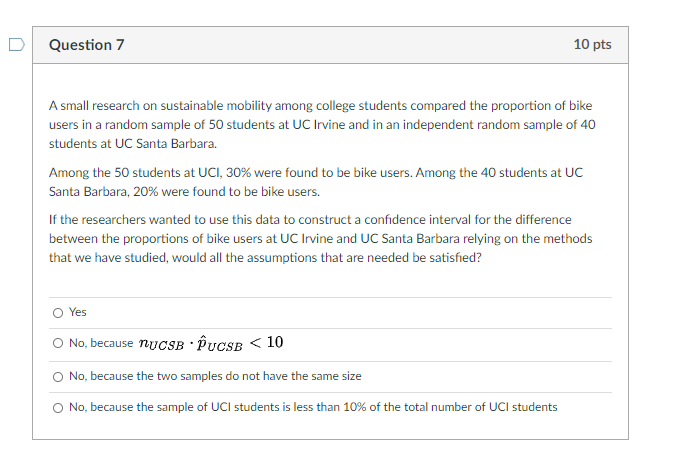# Question Question 7 10 pts A small research on sustainable mobility among college students compared the proportion of bike users in a random sample of 50 students at UC Irvine and in an independent random sample of 40 students at UC Santa Barbara. Among the 50 students at UCI, 30% were found to be bike users. Among the 40 students at UC Santa Barbara, 20% were found to be bike users. If the researchers wanted to use this data to construct a confidence interval for the difference between the proportions of bike users at UC Irvine and UC Santa Barbara relying on the methods that we have studied, would all the assumptions that are needed be satisfied? O Yes O No, because TUCSB UCSB < 10 No, because the two samples do not have the same size O No, because the sample of UCI students is less than 10% of the total number of UCI studentsZ8CZB8 The Asker · Probability and StatisticsTranscribed Image Text: Question 7 10 pts A small research on sustainable mobility among college students compared the proportion of bike users in a random sample of 50 students at UC Irvine and in an independent random sample of 40 students at UC Santa Barbara. Among the 50 students at UCI, 30% were found to be bike users. Among the 40 students at UC Santa Barbara, 20% were found to be bike users. If the researchers wanted to use this data to construct a confidence interval for the difference between the proportions of bike users at UC Irvine and UC Santa Barbara relying on the methods that we have studied, would all the assumptions that are needed be satisfied? O Yes O No, because TUCSB UCSB < 10 No, because the two samples do not have the same size O No, because the sample of UCI students is less than 10% of the total number of UCI students
More
Transcribed Image Text: Question 7 10 pts A small research on sustainable mobility among college students compared the proportion of bike users in a random sample of 50 students at UC Irvine and in an independent random sample of 40 students at UC Santa Barbara. Among the 50 students at UCI, 30% were found to be bike users. Among the 40 students at UC Santa Barbara, 20% were found to be bike users. If the researchers wanted to use this data to construct a confidence interval for the difference between the proportions of bike users at UC Irvine and UC Santa Barbara relying on the methods that we have studied, would all the assumptions that are needed be satisfied? O Yes O No, because TUCSB UCSB < 10 No, because the two samples do not have the same size O No, because the sample of UCI students is less than 10% of the total number of UCI students